### IMO Shortlist 2001 problem G8

Kvaliteta:
Avg: 0.0
Težina:
Avg: 9.0
Let$ABC$ be a triangle with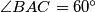$\angle BAC = 60^{\circ}$. Let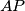$AP$ bisect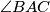$\angle BAC$ and let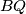$BQ$ bisect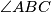$\angle ABC$, with$P$ on$BC$ and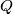$Q$ on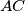$AC$. If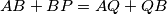$AB + BP = AQ + QB$, what are the angles of the triangle?
Source: Međunarodna matematička olimpijada, shortlist 2001